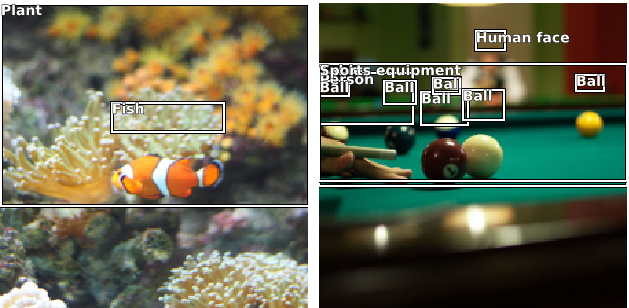# Object Detection - Open Images V5

(Harry Coultas Blum) #1

I’m trying to create an object detection algorithm based on the Google Image Dataset

I’m using the validation set.

The bounding boxes however don’t seem to be in the correct places:Does anyone know what I might be doing wrong here?

2 Likes

#2

Why are you doing the scaling in this cell?

``````labels['XMin'] *= w
labels['XMax'] *= w
labels['YMin'] *= h
labels['YMax'] *= h
``````

I think that’s where your problem comes from. fastai will automatically rescale the bounding boxes for you.

0 Likes

(Harry Coultas Blum) #3

The XMin, XMax, YMin, YMax values are between 0 and 1 and in the examples I saw that they needed to be inline with the actual image width and height.

0 Likes

#4

Ah yes, if it’s 0 to 1 you need this. Have you tried doing it the other way round? I think y is first.

0 Likes

(Harry Coultas Blum) #5

Doing what the other way round? The operations on the DF? Or swapping the order of the values in the labelling function?

`bounds = boxes[['YMin', 'XMin', 'YMax', 'XMax']].values.tolist()`

0 Likes

(Harry Coultas Blum) #6

Just a note that the code works with the tiny coco dataset you show in the documentation but these images are 447x1024. Could that have an effect?

0 Likes

#7

No, the code handles rectangular images. In your case we can see the xs are correct with the fish and the balls, but the ys are improperly scaled, so that’s where there is a problem.

2 Likes

(Harry Coultas Blum) #8

So from the documentation of the dataset

• `XMin` , `XMax` , `YMin` , `YMax` : coordinates of the box, in normalized image coordinates. XMin is in [0,1], where 0 is the leftmost pixel, and 1 is the rightmost pixel in the image. Y coordinates go from the top pixel (0) to the bottom pixel (1).

So it seems that they have the values we need for the `top left bottom right` coordinate system.

0 Likes

(Harry Coultas Blum) #9

Images aren’t all the same size so I had to go through and scale values depending on the size of the image

0 Likes